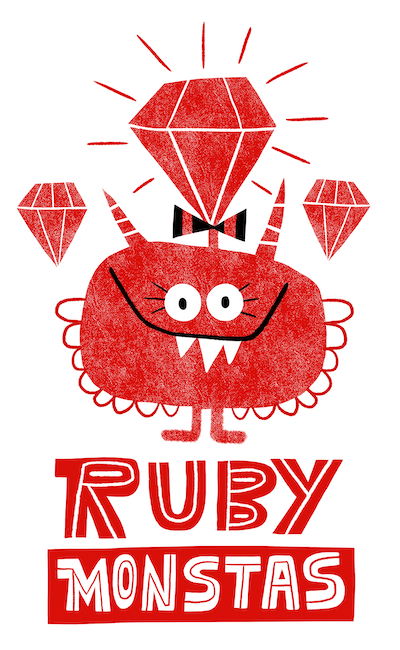# Ruby Monstas

Attribute Writers## What we know so far

For now, we could only input data into an object when initializing it.

## Person class

``````class Person

def initialize(name)
@name = name
end

def name
@name
end

end``````
``````person = Person.new("Jenny")

person.name
# => "Jenny"``````

We can: set the name in the constructor, get the name via name method (reader)

## How would you set a value?

We know how to set a variable value:

``name = "Mary"``

So how could we set a new name on an object?

``person.name = "Mary"``

Let's look at our Person example again:

``````class Person
def initialize(name)
@name = name
end

def name
@name
end
end``````

``````class Person
def initialize(name)
@name = name
end

def name
@name
end

def name=(new_name)
@name = new_name
end
end``````

We add a new method that ends with the "=" sign.

This is the magic so it can be used like a variable assignment.

Technically we would have to call the writer like this:

``person.name=("Mary")``

but Ruby allows us to write it like this:

``person.name = "Mary"``

## Recipe

``````class MyClass
def my_attribute=(new_value)
@my_attribute = new_value
end
end``````

As you can see, the parameter can have any name. The important thing is that we assign the parameter to our instance variable.

## Full Example

``````class Person
def email
@email
end

# this is the writer
def email=(email)
@email = email
end
end``````

Usually though, you keep method names and instance variables equal.

In Ruby, we calls these concepts attribute readers and writers. Most programming languages call them getters and setters.